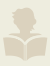# Moonwalking's Reviews > A Tour of the Calculus

by's review

really liked it
bookshelves: math, calculus

Numbers can express real world; that's the assumption.
Let's take the simplest reality, a straight line. Put a zero somewhere and negatives are on the left and positives are on the right. There are large gaps, though, say between zero and one. Now, define a pair of integers (with division in between) as a rational number. We have filled quite a bit, not the whole line, though, because the square root of 2 is not a rational number. Thence come the irrational numbers -- Dedekind's cut is one way to create them. Rationals and irrationals form reals. Now with the irrationals at hand, we have finally been able to assign a number to every point. (don't forget Zeno's paradox)

Two such lines can start forming the two axes of the 2-D plane, etc. But there is no life yet, nothing moves, so far we have just assigned numbers to the points of the world. To bring life and motion, let us connect numbers with one another. Among infinitely many possible relations, we chose a special type, called function, because it appeared to us that this relation is predominant in the world.

One interesting relation is between time and space -- position function. (Let us assume that both can be represented by real numbers.) How much position changes in a period is speed, actually average speed. But what about the speed at this very moment? (Like I speeded over the limit but my average speed was below limit, should I be ticketed?) Ok, let us make the period smaller and smaller and smaller, goes on to infinity -- but never becomes zero, because we cannot divide by zero. We hope that if we start making the period smaller and smaller, the change in position will go closer and closer to a particular numebr, for example like 1.1, 1.01, 1.001, 1.0001, ..., etc. So, we can guess if we could seize the moment, the change in position would have been nothing other than 1 and that is the limit (can you prove it?).

Limit is like trying to know about someone to whom we cannot connect directly, but we have access to close ones of him; and sometimes (for example he is not a weirdo) we could find all there is to find about him just from his near and dear ones -- without ever really meeting him.
The area under the curve is the same idea, accessing something to which we do not have direct access. We can chop the area under the curve into more and more rectangles and hope that if we keep dividing into more and more rectangles the total sum will go closer and closer to a particular number, for example say with 10 rectangles the sum is 1.1, with 100 rectangles it is 1.o1, with 1000 rectangles it is 1.001, etc., again -- not all -- but in some situations (like if the function is continuous) we can say that the exact sum is actually 1 (Can you prove that the sum is exactly 1?).

Speed was differentiation, and area is integration, they have an opposite relationship almost like addition vs. subtraction or multiplication vs. division, one undoes the other. Almost, because if we differentiate a function, we get its derivative -- a single function, but if we integrate (or anti-differentiate) the derivative, we do not get the original function. Instead, we get a family of similar functions which differ by constant. This relation, one undoes the other, is the fundamental theorem of calculus.

Philosophically calculus is the meditation on continuity. Why bother about continuity? Because, motion, that important concept, we feel at some level is continuous. (What about quantum physics?)

Sign into Goodreads to see if any of your friends have read A Tour of the Calculus.